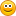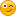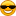## A PRNG for graphics programs?

General FreeBASIC programming questions.
deltarho
Posts: 2530
Joined: Jan 02, 2017 0:34
Location: UK

### Re: A PRNG for graphics programs?

All my PRNGs have a propensity for a uniform distribution, that is flat.

Not being a graphics guy I have a limited imagination with regard the use of a normal distribution. Imagine pushing both ends of a uniform distribution such that the centre starts to lift and the ends start to drop. What we get is a bell shape - a normal distribution.

If we select a range with our uniform distribution then any value within that range will have, in theory, the same likelihood of being selected. However, with a normal distribution there will be bias toward the centre of the range with a lesser chance of the outliers been selected.

Imagine flying in a straight line, and we want a random shift in direction. With a uniform distribution a 10% shift to the left or right is just as likely as a 20% shift to the left or right. With a normal distribution most shifts will be within, say, ± 10% and a further shift to the left or right will be less likely. A shift of ± 60% may be close to impossible.

What I have described may be of no use to a graphics programmer but as intimated above what do I know?

I have added a Gauss function to NR32.bas. I have included it in earlier PRNGs of mine but never went into much detail about its use.

With a range of 5 to 15 we have a mean of 10 and that is what we use in our calculations. The bell shape will be like a fried egg for large standard deviations and a traffic cone for small standard deviations.

This is the formula to use:
value = Mean + (2*(Rnd<0.5)+1)*NR.Gauss*StandardDeviation

If Rnd <0.5 then we subtract from the mean else we add to the mean.

Here are two sets of figures: The first set is the return values from the continuous range function NR.Range(5.,15); the second set is from using the formula above. As you can see the uniform distribution has an even spread whereas the normal distribution sees the 80% likelehood range been compressed and the outliers are not getting a 'look in'. The standard deviation used in the above was 0.78.

Code: Select all

` 5.238640960305929 5.26641181204468 5.441864607855678 6.106326372828335 -> 80% 6.292966627515853 6.482123399619013 6.655931118875742 7.360141410026699 8.295693520922214 8.331588364671916 8.417925206013024 8.784137177281082 8.812430566176772 9.314218556974083 9.48338524904102110.28502926230431 Mid range10.5017472407780610.6935168034397111.205132955219611.5804616478271811.6185927763581312.004227014258512.0470707002095912.2891112347133512.6363455527462112.7179460925981413.1866358267143413.3488451200537413.6938705807551714.19233973370865 -> 80%  8.31719026898374  ---> 95% 8.833664686882646 --> 90% 8.905205663371172 8.984731259750831 9.050552101154359 ->80% 9.188419748145686 9.332126853577261 9.409778093683855 9.594137369002333 9.631111012712989 9.664445172313828 9.670158637957487 9.746191647407478 9.86533963927538310.01657096269018 Mid range10.0806502851545510.2886274555927110.3250679023084210.5010650442889610.5973384135947310.6289167156942210.6333880467268110.9163412868790910.952500909019710.98436492519554 -> 80%11.0564946134344611.24853922263923 --> 90%11.3254319944382711.45563230600347 ---> 95%11.8251349878606`

If this post has any potential for you than add to the opening post:
'Declare Function Gauss() As Double' in Type NR32

Code: Select all

`Private Function NR32.Gauss As DoubleStatic As Long u2_cachedStatic As double u1, u2, x1, x2, w   If u2_cached = -1 Then    u2_cached = 0    Function = u2  Else    Do      x1 = This.RandD      x2 = This.RandD      w = x1 * x1 + x2 * x2    Loop While w >= 1    w = Sqr( -2 * Log(w)/w )    u1 = x1 * w    u2 = x2 * w    u2_cached = -1    Function = u1  End IfEnd Function`

The code has been analysed and the theoretical values of 80%, 90%, 95%, and 99% were calculated as 80.02%, 89.98%, 94.99% and 99.02% so the code is doing a good job. That is not my code by the way and can found on the internet at many sites, for quite a few years now, so I don't know who the original author is. There is some tasty crunching going on there and the throughput on my machine is about 28.3MHz. This is slow compared with Rnd's equivalent but a single value comes in at about 35 nanoseconds, so you will not have the time to put the kettle on.coderJeff
Posts: 3198
Joined: Nov 04, 2005 14:23
Contact:

### Re: A PRNG for graphics programs?

deltarho, neat! I was actually thinking about this just days ago. I did notice posts elsewhere about the slow and thread unsafe nature of fb's PRNG's. What I like most about this approach is that it is simple, can handle multiple instances, and is repeatable. I like that your code highlights the 'Engine' for the LCG; the bit that makes the magic work.
Thank-you.

Last days, I was playing with something similar using a different LCG recipe. Doesn't do any scaling as I was only looking for a 'reasonably' random 8 bit value.

Code: Select all

`type RNDU31   private:      state as ulongint   public:      declare constructor( byval state as ulongint = 1 )      declare function value() as ulong      declare function value256() as ubyteend typeconstructor RNDU31( byval state as ulongint = 1 )   this.state = stateend constructorfunction RNDU31.value() as ulong   state = state * 48271 mod &h7fffffff   return stateend functionfunction RNDU31.value256() as ubyte   return (value \ 256) mod 256end functiondim r as RNDU31 = 1234 '' initialize with seedvar r2 = r             '' make a copyfor i as integer = 1 to 10   print r.value256, r2.value256next`

I have no expertise, so can only assume 'seems OK' until it isn't. The LCG wasn't really the important part. More the form of TYPE and tracking state.
deltarho
Posts: 2530
Joined: Jan 02, 2017 0:34
Location: UK

### Re: A PRNG for graphics programs?

coderJeff wrote:Last days, I was playing with something similar using a different LCG recipe.

That is the Lehmer generator and sometimes referred to as a multiplicative linear congruential generator (MLCG). It works, from a distribution uniformity point of view, but doesn't win any prizes. It should not be used if we want top quality randomness but is OK for a " 'reasonably' random 8 bit value " or "randomish" as I wrote in the opening post. The 'mod &h7fffffff' is hard work but there is a way to take the 'sting' out of that. However, as you wrote "The LCG wasn't really the important part."

It was a good lesson for me because I haven't been giving LCGs the time of day. I have replaced Rnd with NR.RandD in many of the graphics programs published on the forum and none suffered for it. We just have to remember that if randomness is an issue then using a LCG is not a good idea. What I like about PCG32II is that it uses Donald Knuth's 64-bit LCG and then subjects it to some clever conditioning to get us to a 16TB success with PractRand.

coderJeff
Posts: 3198
Joined: Nov 04, 2005 14:23
Contact:

### Re: A PRNG for graphics programs?

Ha. Given your dedication and enthusiasm for the the field, I feel like there should be a 'deltarho scale' for measuring randomness of a PRNG in some magnitude of 'randomish'. If such a thing doesn't exist yet, I feel like you would be the one to create it. Thanks again for sharing your talent.
deltarho
Posts: 2530
Joined: Jan 02, 2017 0:34
Location: UK

### Re: A PRNG for graphics programs?

To have a scale of randomness will require random to have a value. I do not believe that truly random exists in a system governed by quanta. Anyway thanks for the job offer.jj2007
Posts: 1523
Joined: Oct 23, 2016 15:28
Location: Roma, Italia
Contact:

### Re: A PRNG for graphics programs?

coderJeff wrote:Ha. Given your dedication and enthusiasm for the the field, I feel like there should be a 'deltarho scale' for measuring randomness of a PRNG in some magnitude of 'randomish'. If such a thing doesn't exist yet, I feel like you would be the one to create it. Thanks again for sharing your talent.
I echo that request ;-)
deltarho
Posts: 2530
Joined: Jan 02, 2017 0:34
Location: UK

### Re: A PRNG for graphics programs?

@coderJeff

I am being pedantic here but '(value Shr 8) and 255' is over 30% faster than '(value \ 256) mod 256'.
deltarho
Posts: 2530
Joined: Jan 02, 2017 0:34
Location: UK

### Re: A PRNG for graphics programs?

With regard the normal distribution approach the mean is easy to determine but what about the standard deviation? We can, of course, tweak until we get the effect we want; a graphic programmer's lot I should imagine - tweak and view. However, there is a way where we can 'home in' on a standard deviation.

From areas of the standardized normal distribution tables we have.

80% will be within 'Mean ± 1.28155 * Standard_Deviation
90% will be within 'Mean ± 1.64485 * Standard_Deviation
95% will be within 'Mean ± 1.96 * Standard_Deviation
99% will be within 'Mean ± 2.57583 * Standard_Deviation

Suppose we have a range of 5 to 15 and we want 80% between 10 ± 2 then we can write

Mean + 2 = Mean + 1.28155 * Standard_Deviation

Solving that for Standard_Deviation we get

Standard_Deviation = 2/1.28155 = 1.56061

Similarly

90%: Standard_Deviation = 2/1.64485 = 1.215916
95%: Standard_Deviation = 2/1.96 = 1.020408
99%: Standard_Deviation = 2/2.57583 = .776449

So, if we want 80%, between 10 ± 2, with a range 5 to 15, then the Standard_Deviation needs to be 1.566061 (2/1.28155).

Computing 30 times 'Mean + (2*(Rnd<0.5)+1)*NR.Gauss*StandardDeviation' we get

Code: Select all

` 4.619652913527244 6.711644163050348 6.917671371585683 7.557251450179638 7.673496619771295 7.912464745405788 7.934475308016571 8.00700603716532 8/80% 8.444982335639891 8.449249048404639 8.757053707609101 9.109720709136848 9.1164556497201 9.218071315373729 9.224920353894733 9.649653494820951 10.08791660477261  Close to mean 10.12267677615119 10.15411053528741 10.19633606835672 10.23945408199813 10.58389546774586 10.5927239463442 10.71515278965795 10.84199791586124 10.91615306448617 11.39438441406317 11.54395401328038 11.90100821235185 12/80% 12.56033657914575`

If the effect isn't quite right for us then we can simply tweak the standard deviation to change the shape of the bell curve.

If we want 95%, between 0 ± 0.7, with a range -1 to 1, then the Standard_Deviation needs to be 0.357143 (0.7/1.96) and get

Code: Select all

`-1.230975907331296-0.752346780785656-0.7052095746006954 -0.7/95%-0.5588792998238764-0.5322834314140598-0.477609634250348-0.4725738118684049-0.4559794214530061-0.355774311496469-0.3547981252890563-0.2843751370402794-0.2036880409823131-0.2021471460433721-0.1788983789626122-0.1773313794229475-0.08015618698232679 0.02011454290775182 ' Close to mean 0.02806736320243313 0.03525912974679403 0.04491995888051852 0.05478497968893422 0.1335901274849348 0.135610005436726 0.1636206437950888 0.1926416886847802 0.2096077319334332 0.3190228421481434 0.3532430458165068 0.4349338939354888 0.5857824026839209                    0.7/95%`

Simple.deltarho
Posts: 2530
Joined: Jan 02, 2017 0:34
Location: UK

### Re: A PRNG for graphics programs?

I may as well continue.UEZ uses

Code: Select all

`Function RandomRange(fStart As Single, fEnd As Single) As Single  Return Rnd() * (fEnd - fStart) + fStartEnd Function`

In keeping with Microsoft's appending with Ex for their 'posh' versions of APIs my normal distribution version is:

Code: Select all

`Function RandomRangeEx(fStart As Double, fEnd As Double, delta As Double, flag As Double) As Double  ' delta as in mean ± delta. delta/flag is the standard deviation  ' flag is one of the SD definitions  Return (fEnd + fStart)/2 + (2*(Rnd<0.5)+1) * NR.Gauss * delta/flagEnd Function`

Here are a couple of example usages: The first looks at a range -1 to 1 with a delta of 0.7 and an 80% 'capture'; the second looks at a range 20 to 40 with delta of 5 and a 90% 'capture'. We then work out the percentage of values, from 10^8 calls, without the 'capture', that is the less likely to be selected.

Code: Select all

`#include "NR32.bas" #define SD70 1.03643#define SD80 1.28155#define SD90 1.64485#define SD95 1.96#define SD99 2.57583 Function RandomRangeEx(fStart As Double, fEnd As Double, delta As Double, flag As Double) As Double  ' delta as in mean ± delta. delta/flag is the standard deviation  ' flag is one of the SD definitions  Return (fEnd + fStart)/2 + (2*(Rnd<0.5)+1) * NR.Gauss * delta/flagEnd Function Dim As Ulong i, count, n = 10^8For i = 1 to n  x = RandomRangeEx( -1, 1, 0.7, SD80 )  If x < -0.7 Orelse x > 0.7 Then count += 1NextPrint 100*count/n;"%" ' => 19.999275% count = 0For i = 1 to n  x = RandomRangeEx( 20, 40, 5, SD90 )  If x < 25 Orelse x > 35 Then count += 1NextPrint 100*count/n;"%" ' => 10.001282% Sleep`

In UEZ's Fireworks I replaced every RandomRange with RandomRangeEx, except the first one with regard rocketx. I then tried a variety of deltas and SD definitions and was amazed at the scenarios made available some of which 'ruined' the application. However, UEZ will be in a much better position to exploit RandomRangeEx. What I should have done was to replace them one at a time, which I will do. I wrote earlier "What I have described may be of no use to a graphics programmer but as intimated above what do I know?". Well, I now think that RandomRangeEx in the right hands may be a useful tool. Of course some ranges have to be uniformly distributed but for those that don't then a bit of tweaking may be worthwhile.
Last edited by deltarho on Aug 02, 2020 6:49, edited 2 times in total.
deltarho
Posts: 2530
Joined: Jan 02, 2017 0:34
Location: UK

### Re: A PRNG for graphics programs?

As a point of interest instead of the normal distribution we could use the von Mises distribution which, could be argued, is better suited to what we are doing here. It uses a metric called kappa and as kappa tends to zero the distribution tends to the uniform distribution. However, the coding is much more complicated, and I am not sure that it is worth the effort especially if no one has an interest in the normal distribution tool.
dodicat
Posts: 6559
Joined: Jan 10, 2006 20:30
Location: Scotland

### Re: A PRNG for graphics programs?

Here is a skew distribution which could be used to bias something in one way or the other.
Again the random function 4 scores best at bucket filling.

Code: Select all

`randomize 1,4  '<---   4 does bestconst lim=exp(1)#define map(a,b,x,c,d) ((d)-(c))*((x)-(a))/((b)-(a))+(c)#define range(f,l) Rnd*((l)-(f))+(f)#define skewrnd log(range(1,lim))#define Irange(f,l) Int(skewRnd*((l+1)-(f)))+(f)dim as long a(1 to 1000) 'bucketsfor n as long=1 to 50000000    a(Irange(1,1000))+=1 'fill bucketsnext nvar low=a(1),high=a(1000)screen 20for x as long=0 to 1023    var x1=map(0,1023,x,1,1000)     'scale horiz. screen pixels to buckets    var y=map(low,high,a(x1),760,8) 'scale buckets to vert, screen pixels    pset(x,y)next xdim as long count,totfor n as long=1 to 1000-1    if a(n)>a(n+1) then count+=1:tot+= a(n)-a(n+1)'a(n) should be less than a(n+1)next n    locate 5print "  Graph of  Bucket fills"print " low","middle","high"print a(1),a(500),a(1000)print print "overlaps","values"print count,totsleep `
deltarho
Posts: 2530
Joined: Jan 02, 2017 0:34
Location: UK

### Re: A PRNG for graphics programs?

dodicat wrote:Again the random function 4 scores best at bucket filling.

Its randomness is poor but its distribution uniformity is extraordinary.

Would you mind explaining what your code does?

With regard bias the normal distribution tool that I have put forward generates a central bias. The smaller the value of delta and the greater the SDnn value used the greater the bias.
deltarho
Posts: 2530
Joined: Jan 02, 2017 0:34
Location: UK

### Re: A PRNG for graphics programs?

Yours truly wrote:Would you mind explaining what your code does?

I can see what it is doing now.

However, we cannot 'shape' the outcome as we can with delta and SDnn in RandomRangeEx().
dodicat
Posts: 6559
Joined: Jan 10, 2006 20:30
Location: Scotland

### Re: A PRNG for graphics programs?

My distribution is a straight skew, this type of distribution is best by far at filling those tetris shapes I have posted previously.
It might be quite good at other brute force methods in which it is difficult to find a solution by purely ranging through values.
deltarho
Posts: 2530
Joined: Jan 02, 2017 0:34
Location: UK

### Re: A PRNG for graphics programs?

@dodicat

In your Pool program when the computer is playing if a shot can be potted then it gets potted. Suppose we introduced a central bias where a good pot was the most likely but a 'drift' could occur and the larger the drift the less likely that would be.

With a delta/SDnn shaping under user control I could get the computer to play worse than me.This is a type of AI but not artificial intelligence more like artificial incompetence.